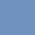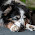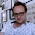PicSaturday, January 3, 2015

Proofs of the Pythagorean Theorem - What Am I Even Trying To Teach?

My students have always struggled to make sense of any sort of proof of the Pythagorean Theorem. It's hard math, but this year I have been trying to push myself to get clearer about why the hard things are hard.

I started making some progress on this when I started asking myself a series of questions during my planning time.
• Why am I good at reconstructing these proofs? What do I know that my students don't?
• If you were good at making sense of these Pythagorean Theorem proofs, what else would you be good at?
After thinking about these two questions, I realized that these visual proofs of the Pythagorean Theorem are part of an entire family of proofs. There are lots of proofs that require the same sort of analysis as these proofs, though they have nothing to do with the Pythagorean Theorem.

This quickly gave my lessons a new life. Here were some implications I drew from this realization:
• In these types of proof, we almost always make progress by describing the same area in two different ways.
• We usually get one of the ways of describing area from thinking about the shape as a whole and a second way by adding up the area of each of its parts.
(By the way, we were working on this activity.)

Figuring out where Pythagorean Theorem proofs exist in the mathematical family tree helped me clarify what I was trying to teach, and that in turn gave me ways to help kids along. I made these worksheets to draw out the connections between the Pythagorean Theorem proofs and other proofs in the "Visual Area Proofs" family.

If you want the files, they're here. I lifted the quadrilateral area problems from here and the dot problems from here.

The takeaway from all this, I think, is that it pays off to get specific about what mathematical knowledge we want our kids to have.

Endnotes:
• Danielson has a similar moment where a student question prompts him to reconsider what's involved in determining the range of a function.
• One of the joys of teaching elementary math is that there's actually a fairly decent specification of what we mean by "fluency with arithmetic."
• This was another instance when directly telling came in helpful. Questioning was definitely important, but it was also important for me to emphasize the whole area/sum of partials parallel. And it was also important for me to encourage students to use this framework when they worked on other Pythagorean Theorem proofs.
• So, what do kids need to know to successfully make sense of these proofs? (1) a geometric interpretation of "a-squared" and so on; (2) a working understanding of the Pythagorean Theorem; (3) knowledge of how these "visual area proofs" work, as detailed above; (4) how to expand (a+b)^2 and do other binomial algebra; (5) how to find the area of triangles and various quadrilaterals. That's why it's hard math -- there's a lot of stuff you need to know, all converging in one problem.

1.I used to show a proof of Pythagoras, but at the stage that students are shown it they actually can't grasp what a proof is. They can do all the steps, sure, but they don't have the ability to put it all together into what it means. So I stopped doing it, and gained back some time to teach them stuff they could grasp.

There's a million things in my life I don't know how they work. Like the clutch on my car or how my wireless router works. Yet I can use them fine. Sometimes learning why things work is a waste of time, because we use them fine without understanding how they work, and we shouldn't fetish proofs for that reason..

My experience then is that proving Pythagoras strikes me as a thing Maths teachers think is important and students don't.

I like proofs, and when students are intellectually mature enough to teach them I enjoy doing proofs with them. But your students may be struggling to make sense of your Pythagoras because they don't yet have the maturity to follow, rather than your inability to frame it correctly.

1.Thanks for the comment, Mark! I'm going to disagree with you, but the points you raise are cogent and interesting.

We both seem to agree that a mature math student should know proofs, and should also be able to make sense of proofs of the Pythagorean Theorem. That's good.

You further argue that though proofs of the PT are important, they aren't important to kids because kids aren't intellectually mature enough to grasp their interest or importance.

What it comes down to, probably, is that I think I can teach my kids to understand these proofs and you don't think that you can. Which is fine. Lots of math is too hard for my students, and maybe it's easier for yours for whatever reason.

My issue is mostly with your argument, which is really an argument against learning anything at all. Students can't figure out the Pythagorean Theorem proofs? They also can't solve equations or divide. There's a million things that we don't understand? Students don't understand algebra or how area works. Math itself tends to be something that Maths teachers find important that students don't.

That said: I agree that we need to decide what's worth our kids' time, and just because something is mathematically important doesn't mean that it's appropriate for our kids. Completely agreed.

2.they aren't important to kids because kids aren't intellectually mature enough to grasp their interest or importance.

No, I think they can be interested, and they can see the importance. I just don't think they can do it.

You can't teach important and interesting philosophical issues to young kids. They don't yet have the mental equipment to balance conflicting concepts of "right", "just" etc. It's why we don't give 16 year olds the vote, for example.

I maintain similarly that few students can properly hold proofs before about 14 or 15 years old. They can follow the steps but they don't get the underlying "why" which is at the heart of proof.

Trying, for example, to get a 12 year old to form a proper proof on their own, even the simplest one, is an exercise in frustration. Yet the same student at 17 can be taught to crush them with ease, and actually enjoy doing so. Clearly at some time their mental equipment changes -- it most certainly is not that they have learned a few more things.

Now we can disagree about when that changeover takes place, sure, but tying to teach things like proofs before that time is more or less useless.

1.Becoming more intellectually mature means they would be interested in Pythagorean theorem and/or feel that they're important? I am unsure about that...

I see everything as an opportunity for the kids to learn. Generally in mathematics, my goal is typically getting them to learning reasoning in general - and not the specifics of how, for example, the Pythagorean theorem works. So, sure, they may not care about the proof of the Pythagorean theorem works, and why would they, but I would get them to think about this process of understanding proofs (or constructing their own) as an exercise in better understanding different ways of reasoning.

3.In case it is useful, this is the simplest example I have seen in the proof-type family you are describing here:
Same areas with pattern blocks.

Since this version doesn't really involve calculating any areas, it was accessible to a 7 year old.

4.Couple of interesting extensions to Pythagoras theorem challenges